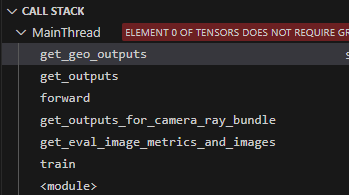When i evaluated my model in which gradients should be computed in forward(), the error occured

``````RuntimeError: element 0 of tensors does not require grad and does not have a grad_fn
``````

The code for the error reporting section is as follows

``````def get_geo_outputs(self, x, feature):
output = self.forward_geo_layers(x, feature)
sdf = output[:, :1]

feature_vectors = output[:, 1:]
outputs=sdf,
inputs=x,
create_graph=True,
retain_graph=True,
only_inputs=True)

``````

And the call stack saved by vscode isthe key functions used for evaluation are

``````    @torch.no_grad()
def get_outputs_for_camera_ray_bundle(self, camera_ray_bundle: RayBundle) -> Dict[str, torch.Tensor]:
"""Takes in camera parameters and computes the output of the model.

Args:
camera_ray_bundle: ray bundle to calculate outputs over
"""
num_rays_per_chunk = 4096   # self.config.eval_num_rays_per_chunk
image_height, image_width = camera_ray_bundle.origins.shape[:2]
num_rays = len(camera_ray_bundle)
outputs_lists = defaultdict(list)
for i in range(0, num_rays, num_rays_per_chunk):
start_idx = i
end_idx = i + num_rays_per_chunk
ray_bundle = camera_ray_bundle.get_row_major_sliced_ray_bundle(start_idx, end_idx)
outputs = self.forward(ray_bundle=ray_bundle)
for output_name, output in outputs.items():  # type: ignore
if not torch.is_tensor(output):
# TODO: handle lists of tensors as well
continue
outputs_lists[output_name].append(output)
outputs = {}
for output_name, outputs_list in outputs_lists.items():
outputs[output_name] = torch.cat(outputs_list).view(image_height, image_width, -1)  # type: ignore
return outputs
``````

and

``````    def get_eval_image_metrics_and_images(self, step: int):
"""This function gets your evaluation loss dict. It needs to get the data
from the DataManager and feed it to the model's forward function

Args:
step: current iteration step
"""
self.model.eval()
image_idx, camera_ray_bundle, batch = self.datamanager.next_eval_image(step)
outputs = self.model.get_outputs_for_camera_ray_bundle(camera_ray_bundle)
metrics_dict, images_dict = self.model.get_image_metrics_and_images(outputs, batch)
assert "image_idx" not in metrics_dict
metrics_dict["image_idx"] = image_idx
assert "num_rays" not in metrics_dict
metrics_dict["num_rays"] = len(camera_ray_bundle)
self.model.train()
return metrics_dict, images_dict
``````

I guess that the error is due to `get_eval_image_metrics_and_images()` calling `self.model.eval()` and the `get_outputs_for_camera_ray_bundle()` with `@torch.no_grad()` context-manager that disabled gradient calculation.

How can i get gradients for other computation when evaluation?Thanks very much for your help

Remove the `@torch.no_grad()` decorator and it should work.